American Journal of Theoretical and Applied Statistics
Volume 5, Issue 5, September 2016, Pages: 280-284

On Properties of the Inverse Cube Transformation of Error Component of the Multiplicative Time Series Model

Dike A. O.1, Otuonye E. L.2,Chikezie D. C.2, Sambo D.1

1Department of Maths and Statistics, AkanuIbiam Federal Polytechnic, Unwana, Afikpo, Nigeria

2Department of Statistics, Faculty of Biological and Physical Sciences, Abia State University, Uturu, Nigeria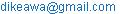(Dike A. O.)

Dike A. O., Otuonye E. L.,Chikezie D. C., Sambo D. On Properties of the Inverse Cube Transformation of Error Component of the Multiplicative Time Series Model. American Journal of Theoretical and Applied Statistics. Vol. 5, No. 5, 2016, pp. 280-284. doi: 10.11648/j.ajtas.20160505.15

Received: May 19, 2016; Accepted: July 1, 2016; Published: August 15, 2016

Abstract: This paper examines the inverse cube transformation of error component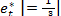of multiplicative time series model. The probability density function (pdf) of the inverse cube root transformation of the multiplicative time series model was established, Further the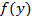was mathematically proved as a proper pdf since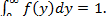The Statistical properties (mean and variance) of the inverse cube transformation were equally shown.

Keywords: Power Transformations, Probability Density Function Error Component, Mean Variance, Multiplicative Time Series

1. Introduction

The cube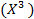transformation is a fairly strong transformation with a substantial effect on distribution shape. It is also used for reducing right skewness, and has the advantage that it can be applied to zero and non negative values. A similar property is possessed by any other root whose power is the inverse of an odd positive integer example 1/3, 1/5, 1/7, etc. The cubic transformation is stronger than the square transformation, though weaker than the logarithm transformation.

Generally, the descriptive time series model is given as: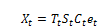(1)

Where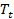is the secular Trend,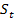is the Seasonal variations,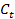is the Cyclical variation and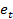is the irregular varions or error component.

In short term series the trend and cyclical components are merged to give the trend-cycle component; hence equation (1) can be rewritten as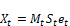(2)

where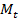is the trend cycle component and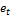are independent identically distributed

Normal errors with meanand variance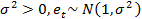2. Data Transformation

In data analysis transformation is the replacement of a variable by a function of that variable, for example, replacing a variable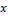by the square root ofor the logarithm of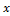. In a stronger sense, a transformation is a replacement that changes the shape of a distribution or relationship. Reasons for transformation include stabilizing variance, normalizing, reducing the effect of outliers, making a measurement scale more meaningful, and to linearize a relationship Iwueze (2011) for more see Bartlett (1947), Box and Cox (1964), Akpanta and Iwueze (2009), Ohakwe et al (2013),etc.

Many time series analysis assume normality and it is well known that variance stabilization implies normality of the series. The most popular and common transformation are the logarithm transformation and the power transformations (square, square root, inverse, inverse square, and inverse square root). A statistical procedure for choice of appropriate data transformation can be obtained in Iwueze et al (2011) and Akpanta et al (2009). It is important to note that, if we apply the inverse cube transformation on model (2), we still obtain a multiplicative time series model given by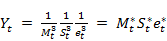(3)

Where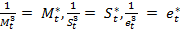Several studies abound in statistical literature on effects of power transformations on the error component of a multiplicative time series model whose error component is classified under the characteristics given in (1). Though not much work has been done on cubic transformation, concentration has been on square transformation. The sole aim of such studies is to establish the conditions for successful transformation. A successful transformation is achieved when the derivable statistical properties of a data set remain unchanged after transformation, the basic properties or assumptions of interest for this study are (i) unit mean and (ii) constant variance. In this end Iwueze (2007) investigated the effect of logarithmic transformation on the error component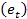of a multiplicative time series model where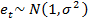and discoursed that the logarithm transform;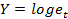can be assumed to be normally distributed with mean, zero and the same variance, σ1< 0.1 also Nwosu et al (2010) and Otuonye (2011) studied the effects of inverse and square root transformation respectively on the error component of the same model. Nwosu et al (2010) discovered that the inverse transform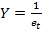can be assumed to be normally distributed with mean, one and the same variance provided,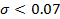similarly Otuonye et al (2011) discovered that the square root transform;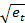can be assumed to be normally distributed with unit mean and variance 4σ2, for σ1< 0.3 where σ12 is the variance of the original error component before transformation.

Ohakwe et al (2012), (2013) studied square root and inverse square root of multiplication error model (MEM) and found the means to be one and variances approximately 4 times of the original error for square root transformation and that the variance for inverse square decreases per unit increase in the shape parameter.

Ibeh et al (2013) studied inverse square transformation of error component of multiplication Time Series Model and found that the mean is one and the increased variance is approximately 4 times the variance of et for σ< 0.07 and losses its symmetry when σ> 0.08.

Ajibade et al (2015) studied the distribution of the inverse square root transformed error component of the multiplicative time series model they found out that the mean is 1.0 and variance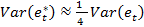when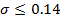Ohakwe et al (2013) gave condition for successful square Transformation in time series modelling, and the result shows that for a successful transformation is σ< 0.027

3. Derivation of the Probability Density Function (Pdf) of Inverse Cube Transformation

Given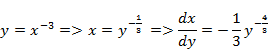So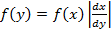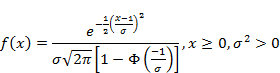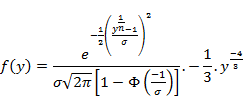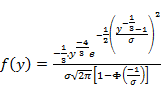(4)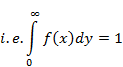We now show that it is a proper pdf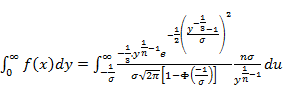(5)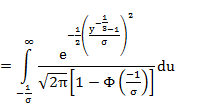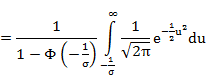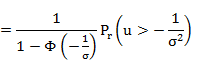But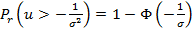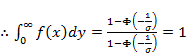(6)

Therefore (8) is a proper pdf.

3.1. Mean for Inverse Cube Transformation

Given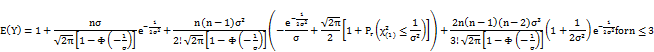For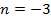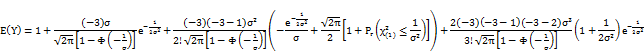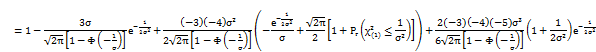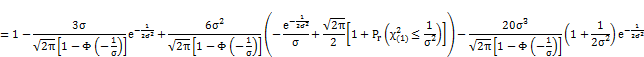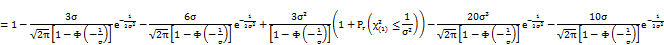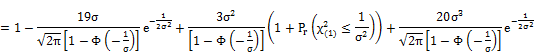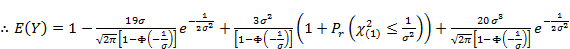(7)

3.2.Variance forInverse CubeTransformation

For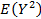Given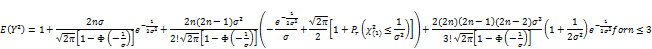For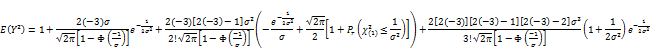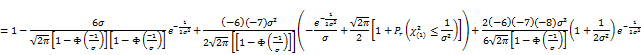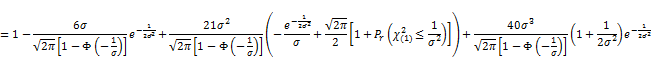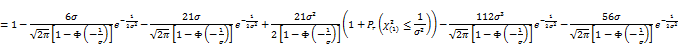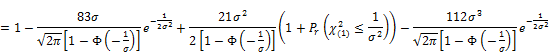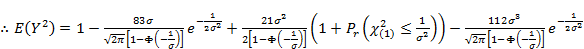(8)

Without any lost in generality, the subsequent terms in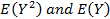with the factor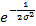will decay fast to zero for values of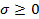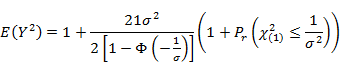And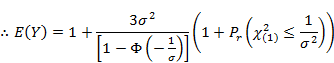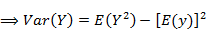(9)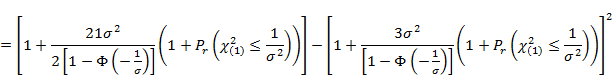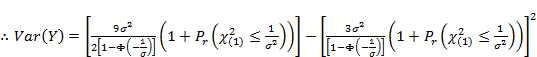(10)

4. Summary and Conclusion

In this study we have established the probability density function of the inverse cube transformation of the error component of the multiplication time series model. The statistical properties (mean and variance) were equally established. The results so far established is a contribution in distribution theory.

References

1. Ajibade B. F, Nwosu C. R and Mbegdu J. I. (2015). The Distribution of the Inverse Square Root Transformed Error Component of The Multiplicative Time Series Model. Journal of Modern Applied Statistical Methods. Vol. 14. Issue 2. pp 171-199.
2. Akpanta A. C. and Iwueze I. S (2009). On Applying the Bartlett Transformation Methods to Time Series Data. Journal of Mathematical Sciences Vol. 20, No. 3. Pp 227-243.
3. Bartlett, M. S. (1947). The use of transformations, Biometrics, 3, 39-52.
4. Box, G. E. P. and Cox, D. R. (1964). An Analysis of Transformations. J. Roy. Statist. Soc., B-26, 2 11-243, discussion 244-252.
5. Chartfield C. (1980). The Analysis of Time Series; An Introduction, Chapman & Hall, London.
6. Ibeh G. C and Nwosu C. R. (2013). Study on the Error Component of Multiplicative Time Series Model under Inverse Square Transformation. American Journal of Mathematics and Statistics (396): pp 362-374.
7. IwuezeIheanyi S. (2007). Some Implications of Truncating the N (1, a2) Distribution to the left at Zero. Journal of Applied Sciences. 7 (2) (2007) pp 189-195.
8. Iwueze I. S, Nwogu E. C., Ohakwe J and Ajaraogu J. C, (201 1). New Uses of Buys-Ballot Table. Journal of Applied Mathematics, 2011, 2. 633-645. DOT: 10.4236/am.2011.25084.
9. Iwueze, I. S., Nwogu, E. C. and Ajaraogu, J. C. (2011). Uses of the Buys-Ballot Table in Time Series Analysis. Journal of Applied Mathematics, 2, 633-645.
10. Iwueze, I. S. and Nwogu, E. C. (2004). Buys-Ballot for Time Series Decomposition. Global Journal of Mathematical Science, 3(2): 83-89.
11. Nwosu C. R, Iwueze I. S. and Ohakwe J. (2010). Distribution of the Error Term of the Multiplicative Time Series Model Under Inverse Transformation. Journal of Advances and Applications in Mathematical Sciences. Volume 7, Issue 2, 2010, pp. 119-139.
12. Ohakwe J, Iwuoha O. and Otuonye E. L (2013). Condition for Successful Square Transformation in Time Series Modeling. Journal of Applied MathematicsVol. 4. pp 680-687.
13. Okereke O. E. and Omekare C. O. (2010) On the Properties of the Truncated Normal Distribution and its Square Root Transformation. Journal of the Nigerian Statistical Association. Vol. 22, 2010, pp 37-43.
14. Osborne, J. (2002). Notes on the Use of Data Transformations, J. Practice Practical Assessment, Research &Evaluation, 8 (6).
15. Otuony E. L, Iwueze I. S. and Ohakwe J. (2011). The Effect of Square Root Transformation on the Error Component of the Multiplicative Time Series Model. International Journal of Statistics and Systems. Vol. 6, No. 4, 2011, pp. 461-476. ISSN: 0973-2675.
16. Ohakwe, J., Dike, O. A. and Akpanta, A. C (2012). The Implication of Square Root Transformation on a Gamma Distribution Error Component of a Multiplicative Time Series Model, Proceedings of African Regional Conference on Sustainable Development, Vol. 6, No. 4, 2012, pp. 65-78.
17. Wei, W. W. (1990). Time Series Analysis: Multivariate Methods. Addision-Wesley Publishing Company, Inc., Redwood City.

 Contents 1. 2. 3. 3.1. 3.2. 4.
Article ToolsAbstractPDF(195K)